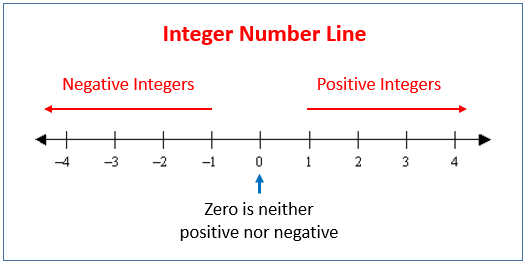# C Program To Check A Number Is Negative, Positive Or Zero | C Programs

C program to check whether a number is negative, positive or zero – In this article, we will detail in on the multiple ways to check whether a number is negative, positive or zero in C programming.

Suitable examples and sample programs have also been added so that you can understand the whole thing very clearly. The compiler has also been added with which you can execute it yourself. In case if you have any doubts related to C Program To Check A Number Is Negative, Positive Or Zero leave a comment here.

The multiple ways used in this piece are as follows:

• Using Standard Method

We all know that the number lines basically differentiates the negative and positive numbers right from the point of zero.

• A negative number is a number that falls to the left of the point of zero on a number line.
• Zero is the middle point of all the negative and positive numbers. It has no absolute value.
• A positive number is a number that falls to the right of the point of zero on a number line.

This is what a number line looks like:As you can see, 0 divides the number line equally into two halves. The numbers on the left are the negative numbers. The numbers on the right are the positive numbers.

The negative numbers have the same absolute value however they have the opposite sign in comparison to the positive numbers.

Zero is considered to be neither Positive nor Negative.

Negative numbers: -5, -17, -2220 etc.

Positive numbers: 3, 7, 222900 etc.

Thus, knowing the same in C programming is as follows:

## Using Standard Method

1. The scanf function reads the entered number n.

2) If n>o then print “it is a positive number”, otherwise print n is a negative number. If n!>0 and n!<0 then print the given number is zero.

Output:

x

## C Program To Count The Total Number Of Notes In A Amount | C Programs

C program to count the total number of notes in a given amount – In ...